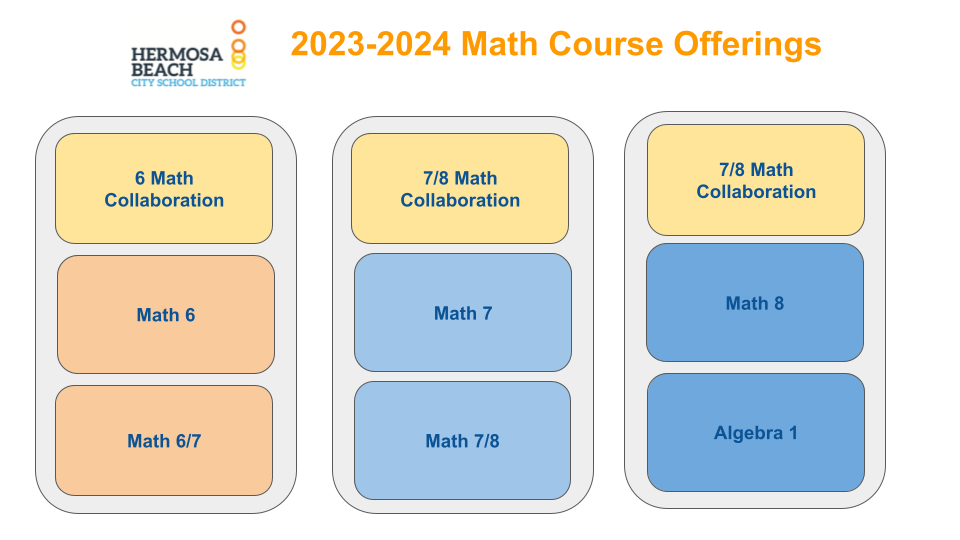# Math

Standards and Frameworks

The California Common Core State Standards for Mathematics include two types of standards: Eight Mathematical Practice Standards (identical for Kindergarten - grade 12) and Mathematical Content Standards (different at each grade level). Together these standards address both the “habits of mind” that students should develop to foster mathematical understanding and the content that students need to know and be able to do.

K-5 Eureka Math

Parent Resources for Eureka Math

Math Skills Support

Middle School Course Offerings